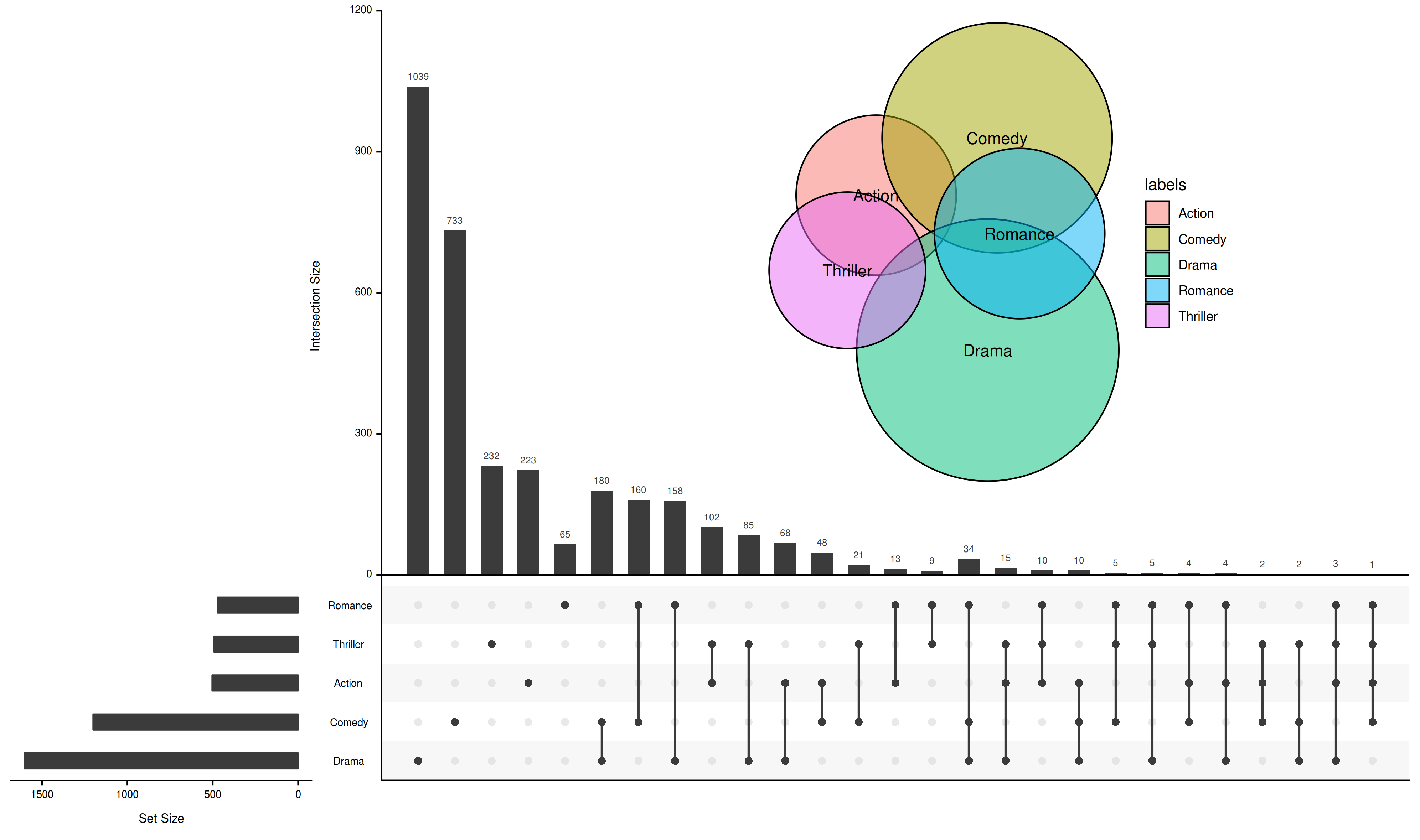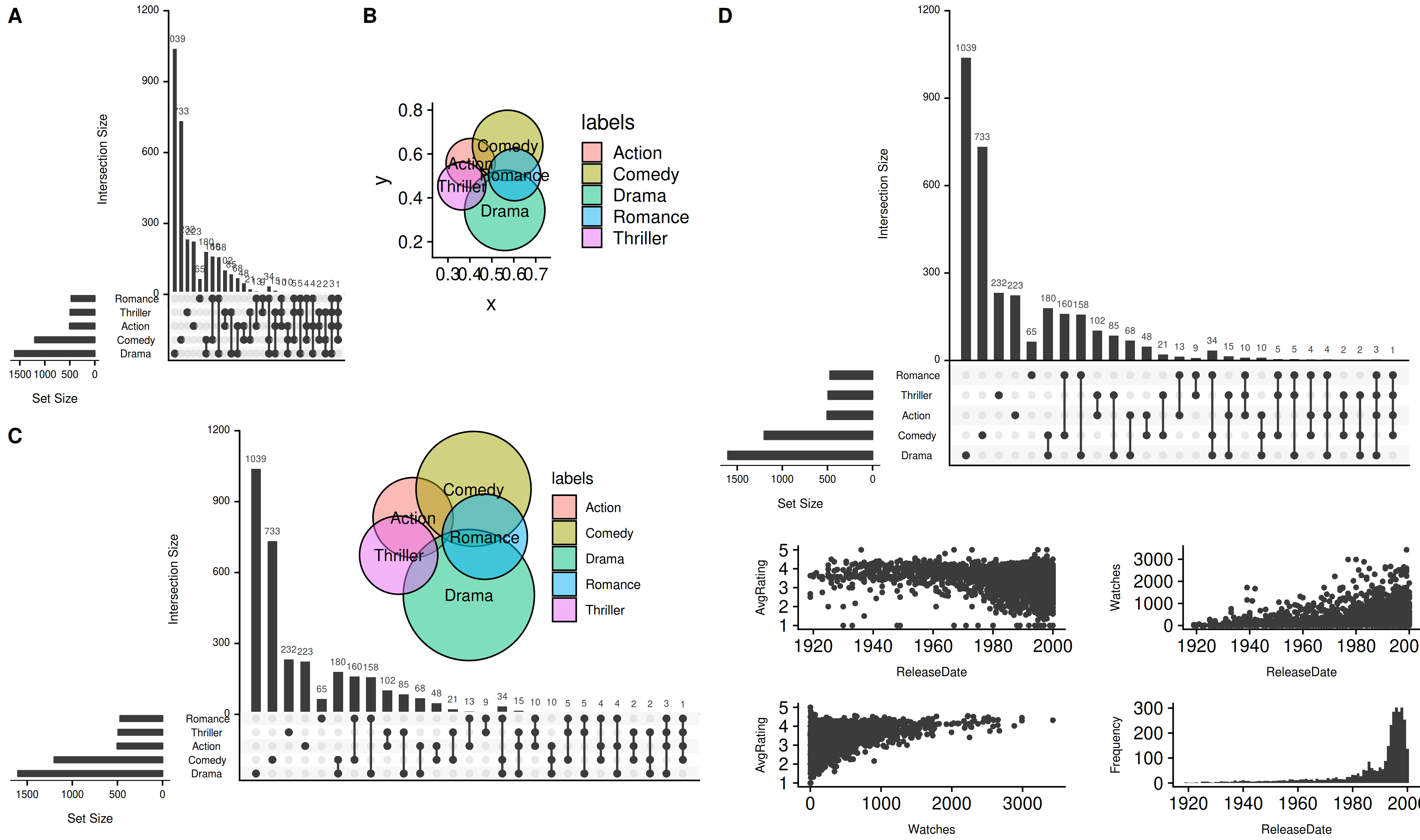``````devtools::install_github("GuangchuangYu/UpSetR")
``````

### UpSetR变ggplot?

``````require(UpSetR)
movies <- read.csv( system.file("extdata", "movies.csv", package = "UpSetR"), header=T, sep=";" )
p1 <- upset(movies)

require(ggplotify)
g1 <- as.ggplot(p1)
``````

### 用熟悉的`ggplot`方式来给UpSet图加注（图）释（层）！

``````require(yyplot)
g2 <- ggvenn(movies[, c(3,6,9,15,17)])

require(ggimage)
g3 <- g1 + geom_subview(subview = g2 + theme_void(), x=.7, y=.7, w=.6, h=.6)
``````### 方便拼图

``````p4 <- upset(movies,attribute.plots=list(gridrows=60,
plots=list(list(plot=scatter_plot, x="ReleaseDate", y="AvgRating"),
list(plot=scatter_plot, x="ReleaseDate", y="Watches"),
list(plot=scatter_plot, x="Watches", y="AvgRating"),
list(plot=histogram, x="ReleaseDate")), ncols = 2)
)

g4 <- as.ggplot(p4)

require(cowplot)

plot_grid(
plot_grid(
plot_grid(g1, g2, ncol=2, labels=c("A", "B")),
g3, ncol=1, labels=c("", "C")
),
g4, ncol=2, labels=c("", "D")
)
```````UpSetR`本身内置所提供的画一些统计图拼在一起的功能，如图D所示，现在有了我们`ggplotify`的转换之后，就显得格外鸡肋，我们可以随意画图，然后拼在一起，突破内置功能的限制。